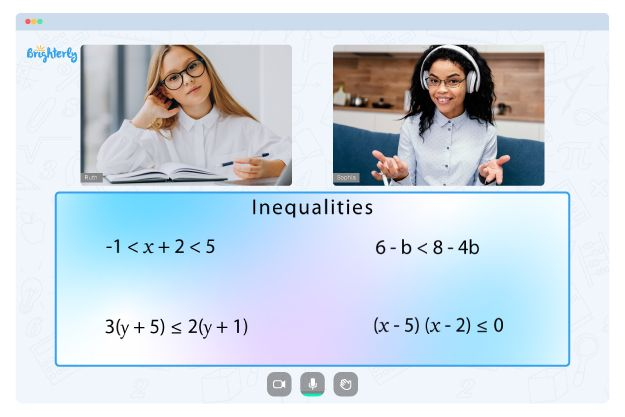# Solving and Graphing Inequalities Worksheets

The solving and graphing inequalities worksheet helps students learn how to solve and graph inequalities. Kids also have access to solving and graphing inequalities worksheet answers that contains related problems.

## Solving Inequalities

To solve an inequality, start by isolating the variable on one side of the inequality sign. Then, use inverse operations (such as addition, subtraction, multiplication, or division) to solve for the variable.

For example, to solve the inequality 2x + 3 < 7, you would subtract 3 from both sides to get 2x < 4, then divide both sides by 2 to get x < 2.

Math for Kids

Is Your Child Struggling With Math?
1:1 Online Math TutoringYou can download our solving systems of inequalities by graphing worksheet PDF to learn how to solve inequalities.

## Graphing Inequalities

To graph the solution to an inequality, you can use a number line. The solution to the inequality x < 2 would be represented by an open dot at 2 on the number line, with everything to the left of 2 being a valid solution.

For example, if it is an equation like x>2 the solution is shown by an arrow that points to the right starting at 2 on the number line. Everything to the right of 2 is a valid solution.

You can download our solving and graphing inequalities pdf to learn how to graph inequalities. You can also download our printable solving and graphing inequalities worksheet answer key pdf to solve practice questions.### Solving and Graphing Inequalities Worksheet PDF

Solving And Graphing Inequalities Worksheet Answer Key Pdf### Solving and Graphing Inequalities Worksheet PDF

Solving And Graphing Inequalities Worksheet Answers### Solving and Graphing Inequalities Worksheet PDF

Solving And Graphing Inequalities Worksheet Pdf### Solving and Graphing Inequalities Worksheet PDF

Solving And Graphing Inequalities Worksheet

Problems with Inequalities?• Start lessons with an online tutor.

Is your child finding it challenging to master inequalities? An online tutor could be the answer.